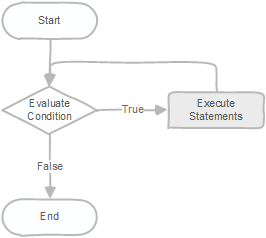# PostgreSQL Tutorial: PL/pgSQL While Loop

August 4, 2023

Summary: in this tutorial, you will learn about the PL/pgSQL `while` loop statement.

## Introduction to PL/pgSQL while loop

The `while` loop statement executes a block of code until a condition evaluates to `false`.

``````[ <<label>> ]
while condition loop
statements;
end loop;
``````

In this syntax, PostgreSQL evaluates the `condition` before executing the `statements`.

If the condition is true, it executes the `statements`. After each iteration, the `while` loop evaluates the `codition` again.

Inside the body of the `while` loop, you need to change the values of some variables to make the `condition` `false` or `null` at some points. Otherwise, you will have an indefinite loop.

Because the `while` loop tests the `condition` before executing the `statements`, the `while` loop is sometimes referred to as a pretest loop.

The following flowchart illustrates the `while` loop statement.## PL/pgSQL while loop example

The following example uses the `while` loop statement to display the value of a counter:

``````do \$\$
declare
counter integer := 0;
begin
while counter < 5 loop
raise notice 'Counter %', counter;
counter := counter + 1;
end loop;
end\$\$;
``````

Output:

``````NOTICE:  Counter 0
NOTICE:  Counter 1
NOTICE:  Counter 2
NOTICE:  Counter 3
NOTICE:  Counter 4
``````

How it works.

• First, declare the `counter` variable and initialize its value to 0.
• Second, use the `while` loop statement to show the current value of the `counter` as long as it is less than 5. In each iteration, increase the value of `counter` by one. After 5 iterations, the `counter` is 5 therefore the `while` loop is terminated.

In this tutorial, you have learned how to use the PL/pgSQL while loop statement to execute a block of code as long as a condition is true.